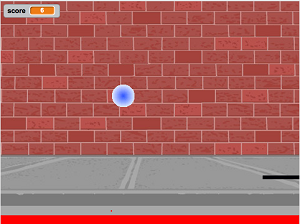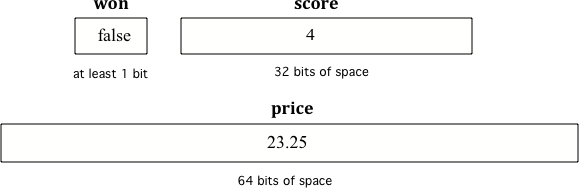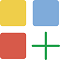# 1.3. Variables and Data Types¶

In this lesson, you will learn about variables and primitive data types.

## 1.3.1. What is a Variable?¶

A variable is a name associated with a memory location in the computer. Computer memory can store a value and that value can change or vary. The following video explains what a variable is and gives a couple of real word examples of variables.

Video: (pHgYlVjagmA)

When you play a game, it will often have a score. Scores often start at 0 and increase. A score can be stored in a variable.Figure 1: A pong game in Scratch with a score shown in the upper left.

## 1.3.2. Data Types¶

There are two types of variables in Java: primitive variables that hold primitive types and object variables that hold a reference to an object of a class. A reference is a way to find the object (like a UPS tracking number helps you find your package). The primitive types on the Advanced Placement Computer Science A exam are:

• int - which store integers (whole numbers like 3, -76, 20393)

• double - which store floating point numbers (decimal numbers like 6.3 -0.9, and 60293.93032)

• boolean - which store Boolean values (either true or false).

`String` is one of the object types on the exam and is the name of a class in Java. A string object has a sequence of characters enclosed in a pair of double quotes - like “Hello”. You will learn more about `String` objects in another unit.

Note

Some languages use 0 to represent false and 1 to represent true, but Java uses the keywords `true` and `false` in boolean variables.

A type is a set of values (a domain) and a set of operations on them. For example, you can do addition with int’s and double’s but not with booleans and Strings.Check your understanding

1-3-1: What type should you use to represent the average grade for a course?

• int
• While you could use an int, this would throw away any digits after the decimal point, so it isn't the best choice. You might want to round up a grade based on the average (89.5 or above is an A).
• double
• An average is calculated by summing all the values and dividing by the number of values. To keep the most amount of information this should be done with decimal numbers so use a double.
• boolean
• Is an average true or false?
• String
• While you can use a string to represent a number, using a number type (int or double) is better for doing calculations.

1-3-2: What type should you use to represent the number of people in a household?

• int
• The number of people is a whole number so using an integer make sense.
• double
• Can you have 2.5 people in a household?
• boolean
• Is the number of people something that is either true or false?
• String
• While you can use a string, a number is better for doing calculations with (like finding the average number of people in a household).

1-3-3: What type should you use to hold the first name of a person?

• int
• People don't usually have whole numbers like 7 as their first name.
• double
• People don't usually have decimal numbers like 3.5 as their first name.
• boolean
• This could only be used if the name was true or false. People don't usually have those as first names.
• String
• Strings hold sequences of characters like you have in a person's name.

1-3-4: What type should you use to record if it is raining or not?

• int
• While you could use an int and use 0 for false and 1 for true this would waste 31 of the 32 bits an int uses. Java has a special type for things that are either true or false.
• double
• Java has a special type for variables that are either true or false.
• boolean
• Java uses boolean for values that are only true or false.
• String
• While you can use a string to represent "True" or "False", using a boolean variable would be better for making decisions.

1-3-5: What type should you use to represent the amount of money you have?

• int
• The integer type (int) can't be used to represent decimal numbers so you couldn't use it if you had any cents.
• double
• The double type can be used to represent an amount of money.
• boolean
• Java uses boolean for values that are only true or false.
• String
• While you can use a string to represent the amount of money you have it is easier to do calculations on the numeric types (int or double).

## 1.3.3. Declaring Variables in Java¶

To create a variable, you must tell Java its data type and its name. Creating a variable is also called declaring a variable. The type is a keyword like int, double, or boolean, but you get to make up the name for the variable. When you create a primitive variable Java will set aside enough bits in memory for that primitive type and associate that memory location with the name that you used.

Computers store all values using bits (binary digits). A bit can represent two values and we usually say that the value of a bit is either 0 or 1. When you declare a variable, you have to tell Java the type of the variable because Java needs to know how many bits to use and how to represent the value. The 3 different primitive types all require different number of bits. An integer gets 32 bits of space, a double gets 64 bits of space and a boolean could be represented by just one bit.Figure 2: Examples of variables with names and values. Notice that the different types get a different amount of space.

To declare (create) a variable, you specify the type, leave at least one space, then the name for the variable and end the line with a semicolon (`;`). Java uses the keyword int for integer, double for a floating point number (a double precision number), and boolean for a Boolean value (true or false).

Here is an example declaration of a variable called score.

```int score;
```

After declaring a variable, you can give it a value like below using an equals sign `=` followed by the value.

```int score;
score = 4;
```

Or you can set an initial value for the variable in the variable declaration. Here is an example that shows declaring a variable and initializing it all in a single statement.

```int score = 4;
```Coding Exercise:

Run the following code to see what is printed. Then, change the values and run it again. Notice that variables are never put into quotes “” because you do not want to print out the variable name, but the value of the variable in memory. If you’re not sure what this means, try putting quotes around the variable and see what happens.

Note

Variables are never put inside quotes (“”) in System.out.print statements. This would print the variable name out letter by letter instead of printing its value.Check Your Understanding

1-3-6: Click on all of the variable declarations in the following code.Variable declarations start with a type and then a name.
```public class Test2
{
public static void main(String[] args)
{
int numLives;
numLives = 0;
System.out.println(numLives);
double health;
health = 8.5;
System.out.println(health);
boolean powerUp;
powerUp = true;
System.out.println(powerUp);
}
}```
1-3-7: Click on all of the variable initializations (first time the variable is set to a value) in the following code.Variables are initialized using name = value;
```public class Test2
{
public static void main(String[] args)
{
int numLives;
numLives = 0;
System.out.println(numLives);
double health = 8.5;
System.out.println(health);
boolean powerUp = true;
System.out.println(powerUp);
}
}```

The equal sign here `=` doesn’t mean the same as it does in a mathematical equation where it implies that the two sides are equal. Here it means set the value in the memory location (box) associated with the name on the left to a copy of the value on the right. The first line above sets the value in the box called score to 4. Also note that the variable has to be on the left side of the `=` and the value on the right. Switching the two is called assignment dyslexia.Coding Exercise: This is an example of assignment dyslexia, when the student has put the value on the left and the declaration on the right side. Try to fix the following code to compile and run.Check Your Understanding

1-3-8: Fill in the following: [blank] age = [blank]; to declare age to be an integer and set its value to 5.

1-3-9: What type should you use for a shoe size like 8.5?

1-3-10: What type should you use for a number of tickets?

Mixed up Code Problems

```        1-3-11: The following code declares and initializes variables for storing a number of visits, a person's temperature, and if the person has insurance or not.  It also includes extra blocks that are not needed in a correct solution.  Drag the needed blocks from the left area into the correct order (declaring numVisits, temp, and hasInsurance in that order) in the right area.  Click on the "Check Me" button to check your solution.int numVisits = 5;
---
Int numVisits = 5; #paired
---
double temp = 101.2;
---
Double temp = 101.2;  #paired
---
boolean hasInsurance = false;
---
Boolean hasInsurance = false; #paired
```

The keyword final can be used in front of a variable declaration to make it a constant that cannot be changed. Constants are traditionally capitalized.

```final double PI = 3.14
```Coding Exercise: Try the following code and notice the syntax error when we try to change the constant PI. Put the comment symbols // in front of that line to remove the error and run it again.

## 1.3.4. Naming Variables¶

While you can name your variable almost anything, there are some rules. A variable name should start with an alphabetic character (like a, b, c, etc.) and can include letters, numbers, and underscores `_`. It must be all one word with no spaces.

You can’t use any of the keywords or reserved words as variable names in Java (`for`, `if`, `class`, `static`, `int`, `double`, etc). For a complete list of keywords and reserved words see http://docs.oracle.com/javase/tutorial/java/nutsandbolts/_keywords.html.

The name of the variable should describe the data it holds. A name like `score` helps make your code easier to read. A name like `x` is not a good variable name in programming, because it gives no clues as to what kind of data it holds. Do not name your variables crazy things like `thisIsAReallyLongName`, especially on the AP exam. You want to make your code easy to understand, not harder.

Note

• Use meaningful variable names!

• Start variable names with a lower case letter and use camelCase.

• Variable names are case-sensitive and spelling sensitive! Each use of the variable in the code must match the variable name in the declaration exactly.

• Never put variables inside quotes (” “).

The convention in Java and many programming languages is to always start a variable name with a lower case letter and then uppercase the first letter of each additional word. Variable names can not include spaces so uppercasing the first letter of each additional word makes it easier to read the name. Uppercasing the first letter of each additional word is called camel case. Another option is to use underscore `_` to separate words, but you cannot have spaces in a variable name.

Java is case sensitive so `playerScore` and `playerscore` are not the same. Run the code below to see the difference.Check Your Understanding

1-3-12: What is the camel case variable name for a variable that represents a shoe size?

1-3-13: What is the camel case variable name for a variable that represents the top score?

## 1.3.5.Debugging Challenge : Weather Report¶

Debug the following code. Can you find the all the bugs and get the code to run?

## 1.3.6. Summary¶

• A variable is a name for a memory location where you can store a value that can change or vary.

• A variable can be declared and initialized with the following code:

```int score;
double gpa = 3.5;
```
• Data types can be categorized as either primitive type (like int) or reference type (like String).

• The three primitive data types used in this course are int (whole numbers), double (decimal numbers), and boolean (true or false).

• Each variable has associated memory that is used to hold its value.

• The memory associated with a variable of a primitive type holds an actual primitive value.

• When a variable is declared final, its value cannot be changed once it is initialized.